# Ohms Law

## Ohms Law

A term must now be introduced which is: electrical resistance or just resistance.

Resistance is a force that opposes the flow of electric charge, measured in ohms (Ω). Resistance in a wire is caused by the collision between the electrons (charge carriers) and the ions in the wire.

Ohmic resistors obey Ohm’s law which states: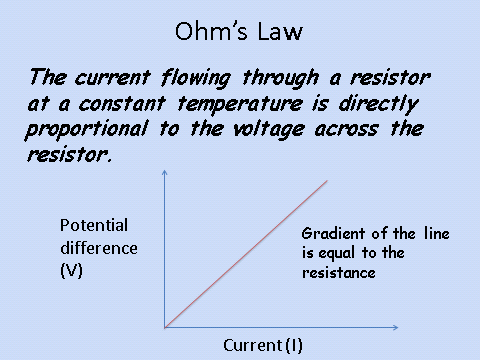Note: You may have heard potential difference be described as voltage. The exam will always ask using the term potential difference, but you gain credit for using either potential difference or voltage in your answers.

## Equation

The equation which you need to recall and apply is:

Potential difference = current x resistance

This can also be written as:

V = I x R

• V = potential difference, volts (V)
• I = current, amps (A)
• R = resistance, ohms (Ω)

## Resistance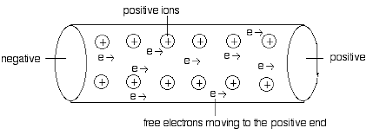Resistance occurs due to the collision between the electrons and the ions in the wire. These collisions reduce the rate of flow and therefore the current is decreased.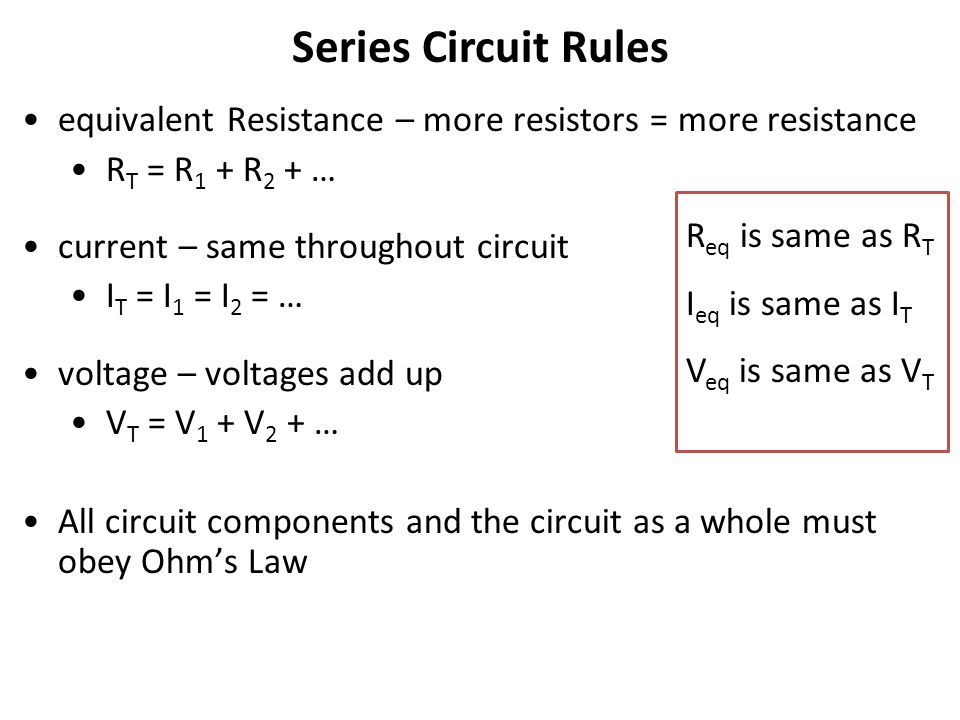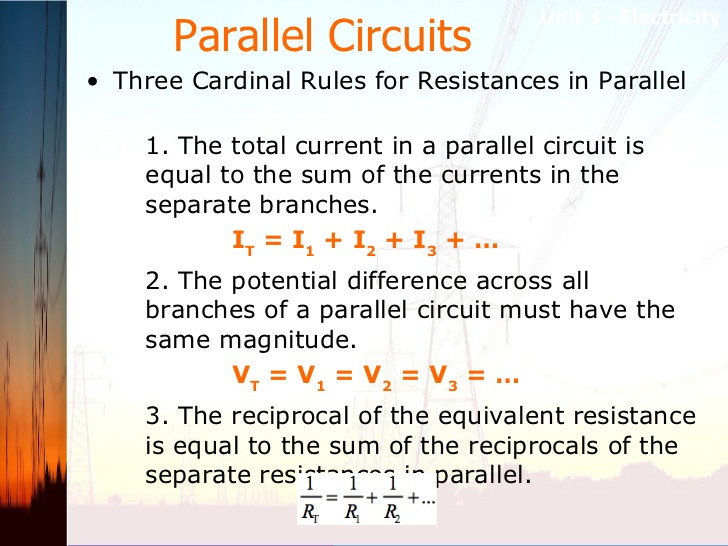Resistance in a parallel circuit is lower than a series circuit. This is due to the fact that the branches of a parallel circuit have a reduced current. This means there are fewer collisions with the ions in the wires.

Try this: what is the potential difference across the 3Ω resistor?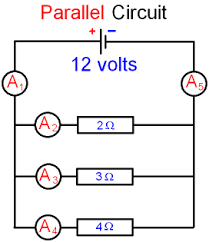Answer: 12V

The voltage across a lamp is 12 V and the current through the lamp is 2 A. What is the resistance of the lamp?
6 Ohms
The resistance of a thermistor is 34 Ω and the current through it is 0.3 A. What is the voltage across the thermistor?
10.2V
The resistance of an ipod shuffle is 3 kΩ and the current through it is 4 mA. What is voltage of its power source?
12V
What is the rule for potential difference in a series circuit?
potential difference adds up
What is the difference between a series and parallel circuit?
Your answer should include: Series one loop / parallel multiple